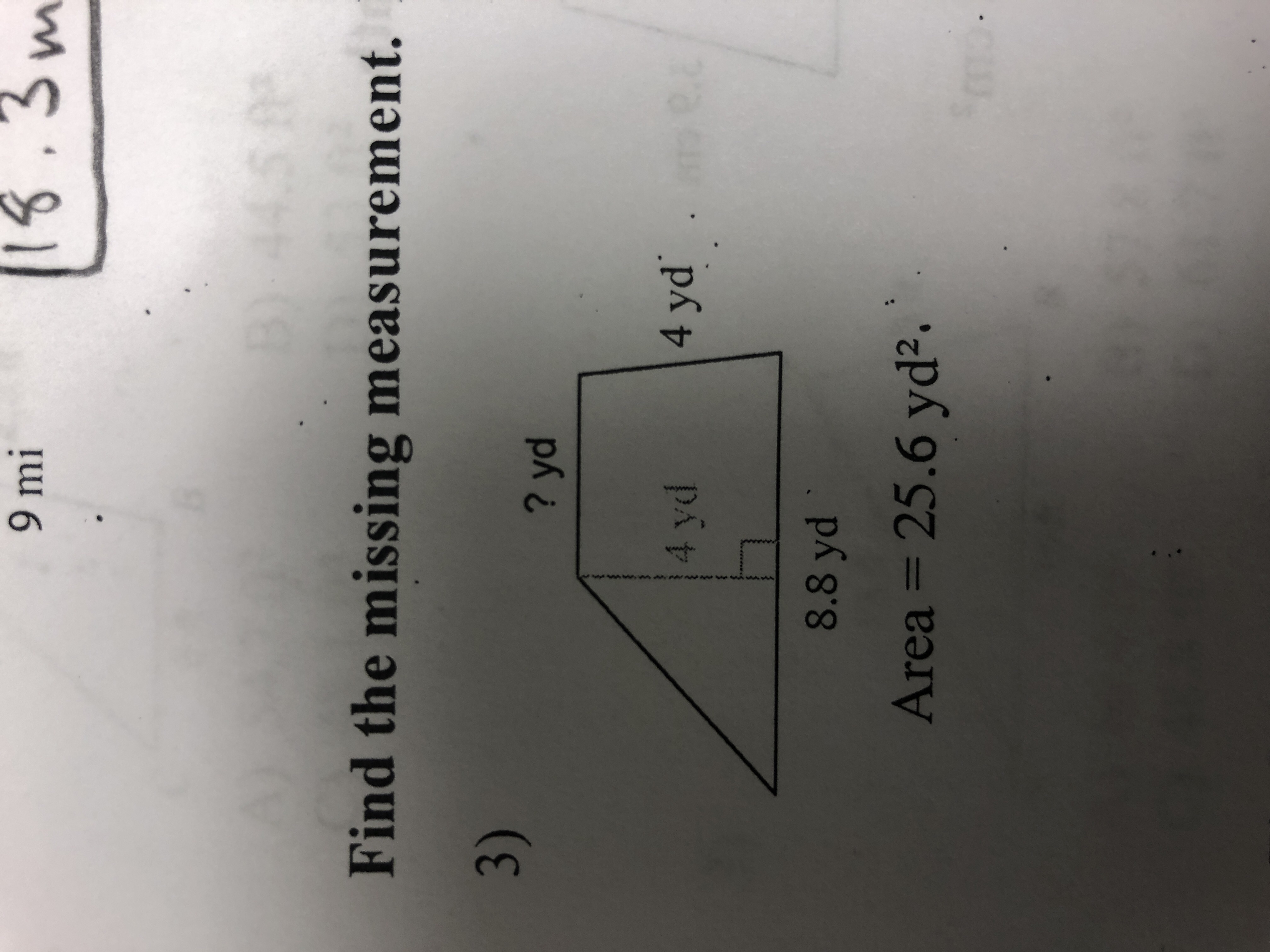# 1.39 miFind the missing measurement.3)? yd4 yd8.8 ydArea 25.6 yd2

Question
28 viewshelp_outlineImage Transcriptionclose1.3 9 mi Find the missing measurement. 3) ? yd 4 yd 8.8 yd Area 25.6 yd2 fullscreen
check_circle

Step 1

Here the total area of the below diagram is given as 25.6 yd2. Let us assume the missing side DE as x yd. the length of total base AC is given as 8.8 yd. So BC is equal to x yd and AB is (8.8-x) yd.

The total area = area of traiangle ABE + Area of BCDE

Step 2

Here let us compute area of  ABE and area of BCDE. Euate their sum to total given area and compute the missing side.

Step 3

the area of a right angled triangle ABE is calculated by the belo...

### Want to see the full answer?

See Solution

#### Want to see this answer and more?

Solutions are written by subject experts who are available 24/7. Questions are typically answered within 1 hour.*

See Solution
*Response times may vary by subject and question.
Tagged in

### Algebra